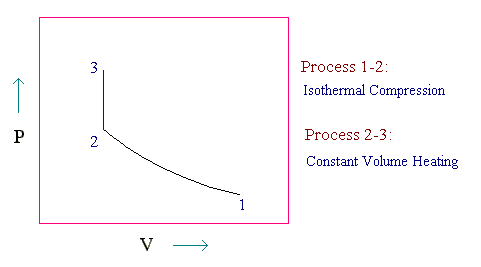### Isothermal-Isochoric Process

One mole of an ideal gas, initially at 20oC and 1 bar, undergoes the following mechanically reversible changes. It is compressed isothermally to a point such that when it is heated at constant volume to 100oC its final pressure is 10 bar. Calculate Q, W, DU, and DH for the process. Take Cp = 7R/2 and Cv = 5R/2.

Calculations:

The given process is represented in PV diagram as follows:We can find the pressure and volume of points 1, 2 and 3 by equation of state (ideal gas equation)

For one mole of ideal gas,

P1V1 = RT1

P2V2 = RT2

P3V3 = RT3

For the given process, T2 = T1 and V3 = V2.

V1 = R x (273 + 20)/1 = 293R

And V3 = R x (273 + 100) / 10 = 37.3R

For the point 2, P2 = R x (273 + 20) / (37.3R) = 293/37.3 = 7.855 bar

PVT conditions of the points 1,2 and 3's are:

 Point P, bar V T, Kelvin 1 1 293R 293 2 7.855 37.3R 293 3 10 37.3R 373

For the isothermal process:

DU = 0

DH = 0

W = ò PdV = ò RT dV/V = RT ln (V2/V1) = R x 293 x ln (37.3/293) = -604R

Q = -W = 604R (since Q + W = DU)

Work is done on the system and heat is given out by the system

For the constant volume heating (isochoric process):

W = 0

DU = Cv(T3 - T2) = (5R/2) x (373 - 293) = 200R

DH = Cp(T3 - T2) = (7R/2) x (373 - 293) = 280R

Q = DU - W = 200R

Summary:

 Process Q (heat given to the system) W (work done on the system) DU DH Isothermal compression -604R = -604 x 8.314 = -5021.66 J 604R = 5021.66 J 0 J 0 J Constant volume heating 200R = 1662.8 J 0 J 1662.8 J 280R = 2327.92 J

HOME